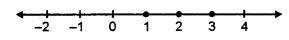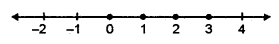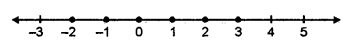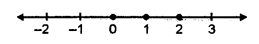## ML Aggarwal Class 7 Solutions for ICSE Maths Chapter 9 Linear Equations and Inequalities Ex 9.3

Question 1.
If the replacement set is (- 5, – 3, – 1, 0, 1, 3, 4), find the solution set of:
(i) x < -2
(ii) x > 1
(iii) x ≥ -1
(iv) -5 < x < 3
(v) -3 ≤ x < 4
(vi) 0 ≤ x < 7.
Solution:
Replacement set = {-5, -3, -1, 0, 1, 3, 4}
(i) Solution set of x < – 2 = {-5, -3}
(ii) Solution set of x > 1 = {3, 4}
(iii) Solution set of x ≥ -1 = {- 1, 0, 1, 3, 4}
(iv) Solution set of -5 < x < 3 = {-3, -1, 0, 1}
(v) Solution set of -3 ≤ x < 4 = {-3, -1,0, 1, 3}
(vi) Solution set of 0 ≤ x < 7 = {0, 1, 3, 4}

Question 2.
Represent the following inequations graphically:
(i) x ≤ 3, x ∈ N
(ii) x < 4, x ∈ W
(iii) -2 ≤ x < 4, x ∈ I
(iv) -3 ≤ x ≤ 2, x ∈ I
Solution:
(i) Given x ≤ 3, x ∈ N
The solution set = {1, 2, 3}
The solution set is shown by thick dots on the number line.(ii) x < 4, x ∈ W
The solution set = {0, 1, 2, 3}
The Solution set is shown by thick dots on the number line.(iii) -2 ≤ x < 4, x ∈ I
The solution set = {-2, -1, 0, 1, 2, 3}
The graph of the solution set is shown by thick dots on the number line.(iv) -3 ≤ x ≤ 2, x ∈ I
The solution set = {-3, -2, -1, 0, 1, 2}
The graph of the solution set is shown by thick dots on the number line.Question 3.
Solve the following inequations.
(i) 4 – x > -2, x ∈ N
(ii) 3x + 1 ≤ 8, x ∈ W
Also represent their solutions on the number line.
Solution:
(i) Given, 4 – x > -2
Subtract 4 from both sides
⇒ -4 + 4 – x > -2 – 4 – x > -6
⇒ x < 6 (Reverse the symbols)
As x ∈ N, the solution set = {1, 2, 3, 4, 5}
The graph of the solution set(ii) Given 3x + 1 ≤ 8.
Subtracting -1 from both sides,
3x + 1 – 1 ≤ 8 – 1
3x ≤ 7
Dividing both sides by 3
⇒ x ≤ $$\frac { 7 }{ 3 }$$
As x = W, the solution set = {0, 1, 2}
The graph of the solution setQuestion 4.
Solve 3 – 4x < x – 12, x ∈ {-1, 0, 1, 2, 3, 4, 5, 6, 7}.
Solution:
Given 3 – 4x < x – 12
Subtracting 3 from both sides
⇒ -3 + 3 – 4x < x – 12 – 3
⇒ -4x < x – 15
Subtracting x from both sides
⇒ -4x – x < x – x – 15
⇒ -5x < -15 ⇒ x > 3
(Dividing by – 5 and reverse the symbols)
As x ∈ {-1, 0, 1, 2, 3, 4, 5, 6, 7}
The solution set = {4, 5, 6, 7}

Question 5.
Solve -7 < 4x + 1 ≤ 23, x ∈ I.
Solution:
Given, -7 < 4x + 1 ≤ 23.
We take -7 < 4x + 1 ≤ 23.
Subtracting -1 from all sides,
-7 – 1 < 4x + 1 – 1 ≤ 23 – 1
-8 < 4x ≤ 22
$$\frac { -8 }{ 4 }$$ < $$\frac { 4x }{ 4 }$$ ≤ $$\frac { 22 }{ 4 }$$ (Dividing by 4)
-2 < x ≤ 5.5
As x ∈ I, the solution set = {-1, 0, 1, 2, 3, 4, 5}.